• 累计撰写 276 篇文章
• 累计收到 33 条评论

# 天天说AI，那么AI能帮我们干什么？2023-05-06 / 0 评论 / 53 阅读 / 正在检测是否收录...

AI已经侵入现实生活中的方方面面。定个闹钟，预定电影票，叫外卖等等，更别说写个代码了
AI尤其会写代码，究其原因：AI本身就是代码构成的，AI当然更懂代码！

1.对话型AI不能帮助整理EXCEL，所以我们需要叫AI帮写成代码。excel能用的代码通常是VBA，这里我们的需求就是：请帮我按照描述写一段VBA代码
2.我们的数据源是什么？在这里数据源就是EXCEL表单
3.表单里面有什么表？表单有Sheet1和Sheet2表
4.核对Sheet1和Sheet2表的那些数据？核对Sheet1和Sheet2的A,B,C列的每一行
5.是否需要生成合并后的表单？这里不是必选项，请根据自己的具体需求告诉AI，请一定要逻辑清楚，条理简单明了。我选择是：将Sheet1 A,B,C列的值复制到Sheet3的A,C,E列的对应行,将Sheet2 A,B,C列的值复制到Sheet3的B,D,F列的对应行
6.核对条件是？如果Sheet1和Sheet2的A,B,C列的的对应行的值都相等，则Sheet3的G列对应的行填写OK，否则Sheet3的A,B,C,D,E,F列的对应行使用红颜色标注不相等的值

Sub compareSheets()
Dim sheet1 As Worksheet
Set sheet1 = ThisWorkbook.Worksheets(“Sheet1”)
Dim sheet2 As Worksheet
Set sheet2 = ThisWorkbook.Worksheets(“Sheet2”)
‘ 检查需要处理的两个工作表是否存在
If sheet1 Is Nothing Or sheet2 Is Nothing Then
MsgBox “Sheet1 或 Sheet2 工作表不存在。”
Exit Sub
End If
Dim sheet3 As Worksheet
sheet3.Name = “Sheet3”
‘ 设定 Sheet3 的 A-G 列标题
sheet3.Range(“A1:G1”).Value = Array(“Sheet1-A”, “Sheet2-A”, “Sheet1-B”, “Sheet2-B”, “Sheet1-C”, “Sheet2-C”, “Result”)
‘ 对 Sheet1 和 Sheet2 的每一行逐行处理，并将结果存储到Sheet3中
Dim row_index As Integer
row_index = 2
Do While sheet1.Cells(row_index, 1).Value <> “” And sheet2.Cells(row_index, 1).Value <> “”
If sheet1.Cells(row_index, 1).Value = sheet2.Cells(row_index, 1).Value And _
sheet1.Cells(row_index, 2).Value = sheet2.Cells(row_index, 2).Value And _
sheet1.Cells(row_index, 3).Value = sheet2.Cells(row_index, 3).Value Then
‘ 如果A,B,C三列相同，取出 Sheet1 和 Sheet2 相同行的值并复制到 Sheet3 的对应列
sheet3.Cells(row_index, 1).Value = sheet1.Cells(row_index, 1).Value ‘ Sheet1 A
sheet3.Cells(row_index, 3).Value = sheet1.Cells(row_index, 2).Value ‘ Sheet1 B
sheet3.Cells(row_index, 5).Value = sheet1.Cells(row_index, 3).Value ‘ Sheet1 C
sheet3.Cells(row_index, 2).Value = sheet2.Cells(row_index, 1).Value ‘ Sheet2 A
sheet3.Cells(row_index, 4).Value = sheet2.Cells(row_index, 2).Value ‘ Sheet2 B
sheet3.Cells(row_index, 6).Value = sheet2.Cells(row_index, 3).Value ‘ Sheet2 C
‘ 在 Sheet3 的 G 列填入 OK
sheet3.Cells(row_index, 7).Value = “OK”
Else
‘ 取出 Sheet1 和 Sheet2 不相等的值并将对应的单元格设置为红色
sheet3.Cells(row_index, 1).Value = sheet1.Cells(row_index, 1).Value ‘ Sheet1 A
sheet3.Cells(row_index, 3).Value = sheet1.Cells(row_index, 2).Value ‘ Sheet1 B
sheet3.Cells(row_index, 5).Value = sheet1.Cells(row_index, 3).Value ‘ Sheet1 C
sheet3.Cells(row_index, 2).Value = sheet2.Cells(row_index, 1).Value ‘ Sheet2 A
sheet3.Cells(row_index, 4).Value = sheet2.Cells(row_index, 2).Value ‘ Sheet2 B
sheet3.Cells(row_index, 6).Value = sheet2.Cells(row_index, 3).Value ‘ Sheet2 C
sheet3.Cells(row_index, 1).Font.Color = RGB(255, 0, 0) ‘ 如果有不同，将当前行标注红色
sheet3.Cells(row_index, 2).Font.Color = RGB(255, 0, 0)
sheet3.Cells(row_index, 3).Font.Color = RGB(255, 0, 0)
sheet3.Cells(row_index, 4).Font.Color = RGB(255, 0, 0)
sheet3.Cells(row_index, 5).Font.Color = RGB(255, 0, 0)
sheet3.Cells(row_index, 6).Font.Color = RGB(255, 0, 0)
End If
row_index = row_index + 1
Loop
End Sub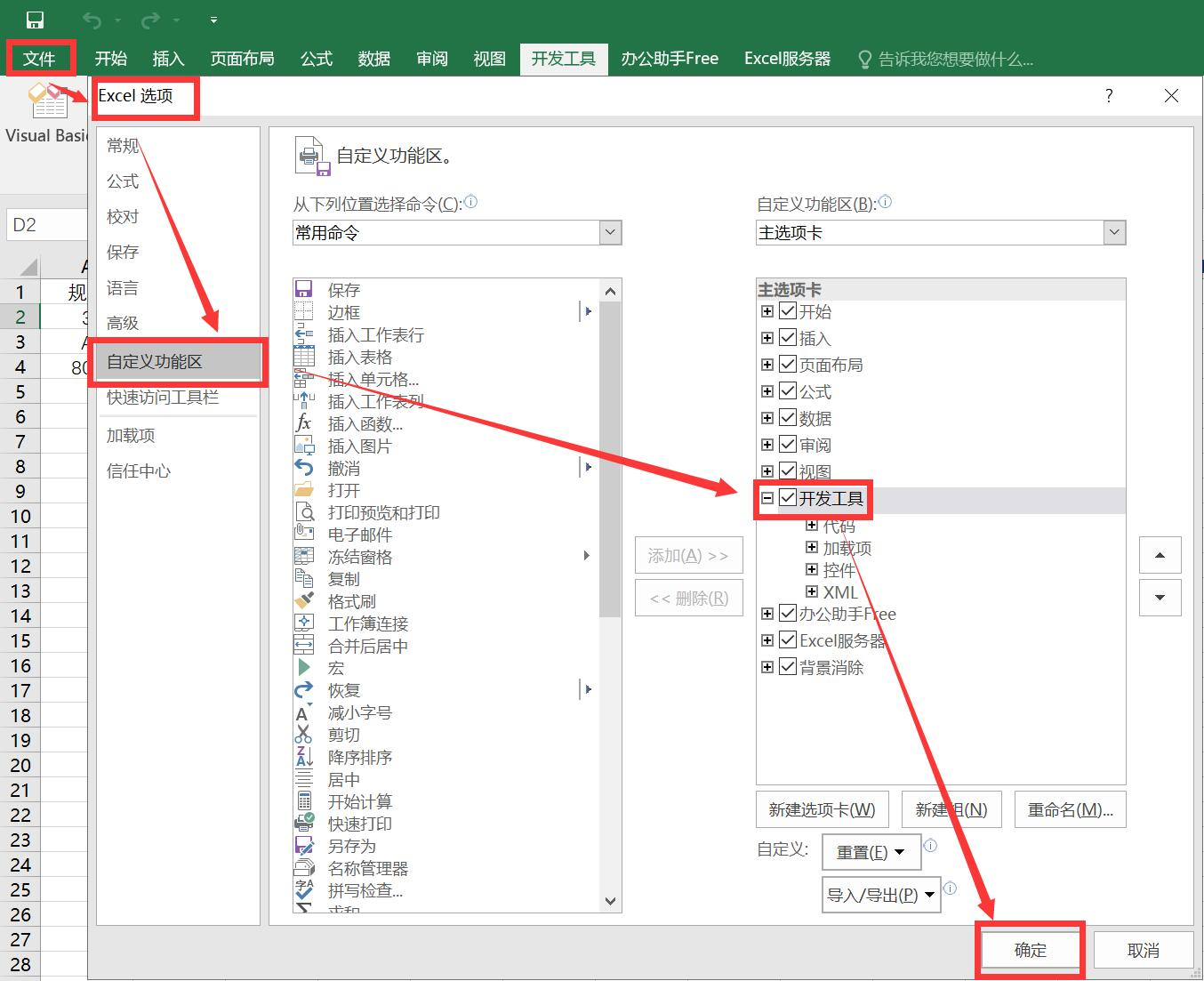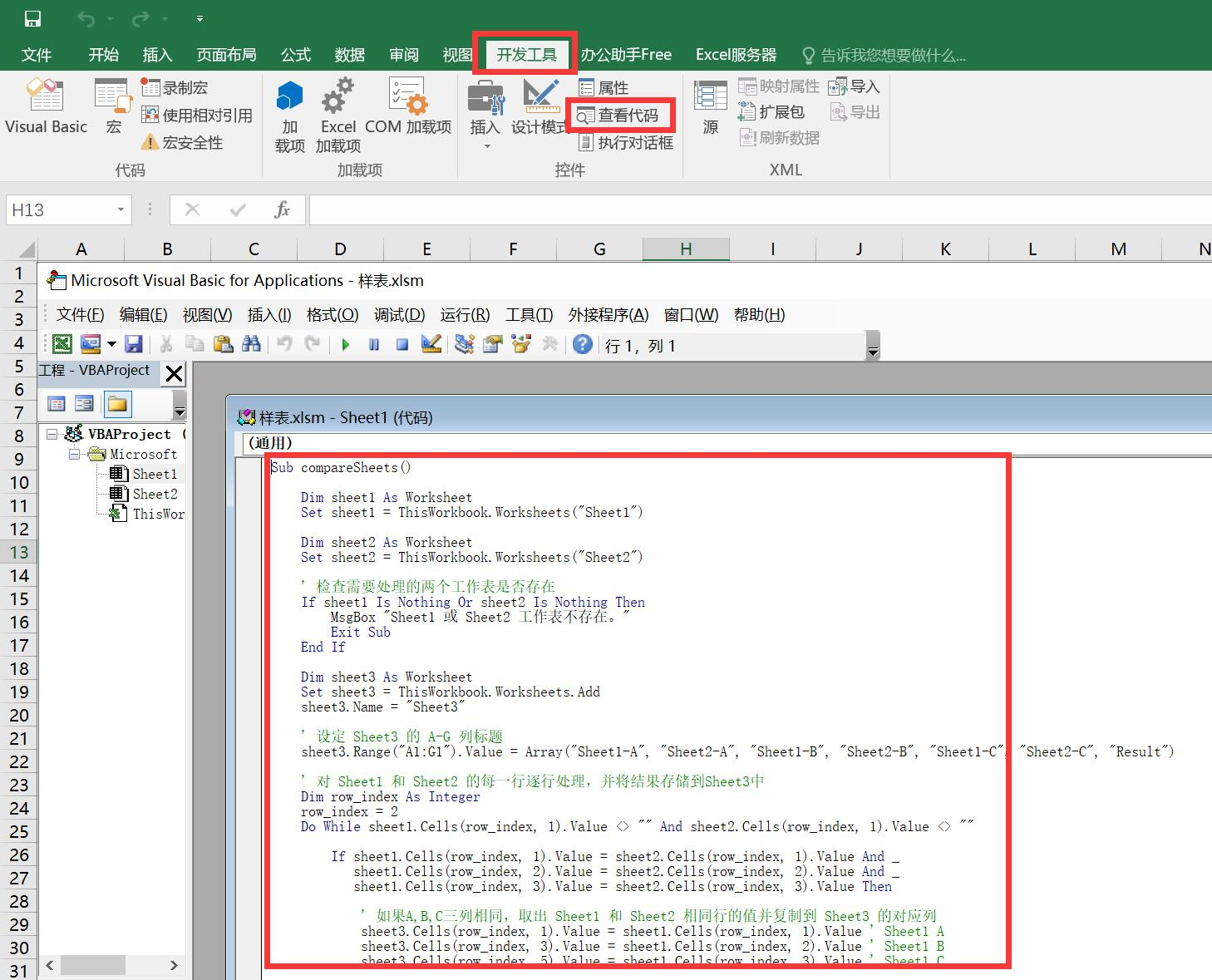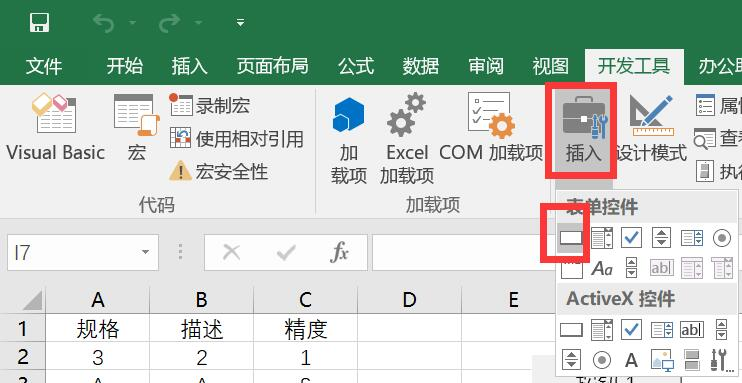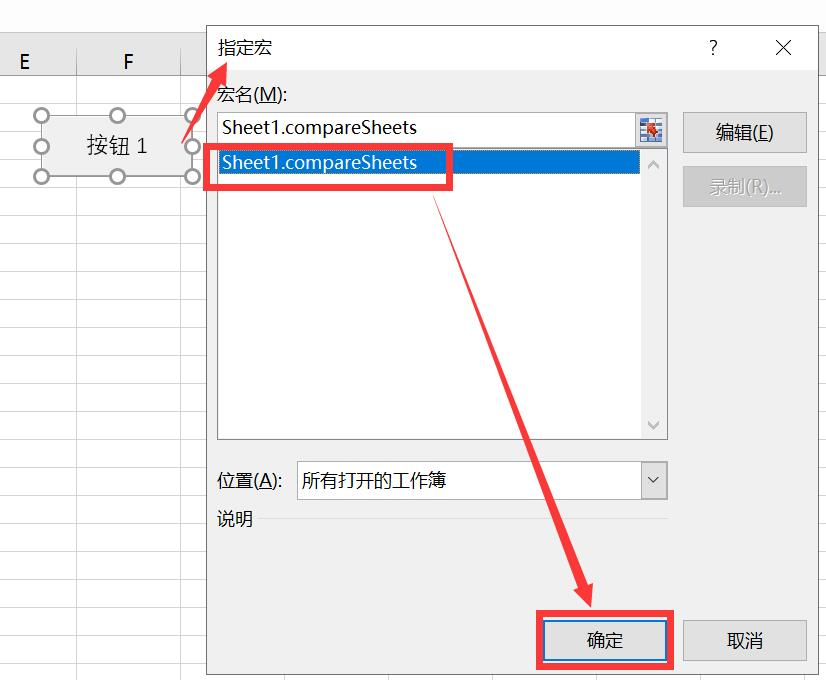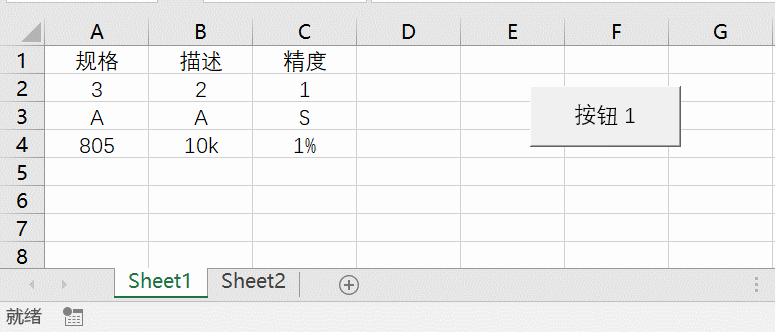1